# 1. Write a Matlab function, piecewise (), that will calculate and return the value of the...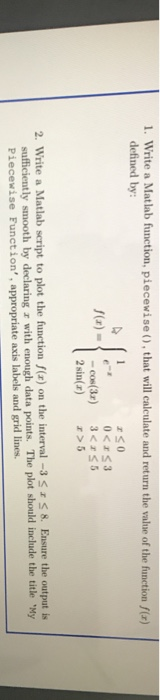1. Write a Matlab function, piecewise (), that will calculate and return the value of the function f(x) defined by: 0

piecewise.m

function y=piecewise(x)
if(x<=0)
y=1;
elseif(x>0 && x<=3)
y=exp(-x);
elseif(x>3 && x<=5)
y=-cos(3*x);
elseif(x>5)
y=2*sin(x);
end
end

plotpiecewise.m

x=[];
for i=-3:0.01:8
x=[x,i];
end
y=[];
for i=-3:0.01:8
y=[y,piecewise(i)];
end
plot(x,y);
xlabel('x')
ylabel('y')
title('y=piecewise(x)')

Output Plot: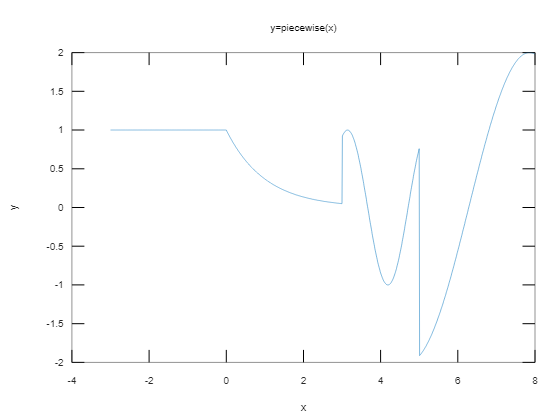##### Add Answer of: 1. Write a Matlab function, piecewise (), that will calculate and return the value of the...
Similar Homework Help Questions
• ### 1. Write a short MATLAB script or function (m-file) that generates the functions (t)eu(t) for a =...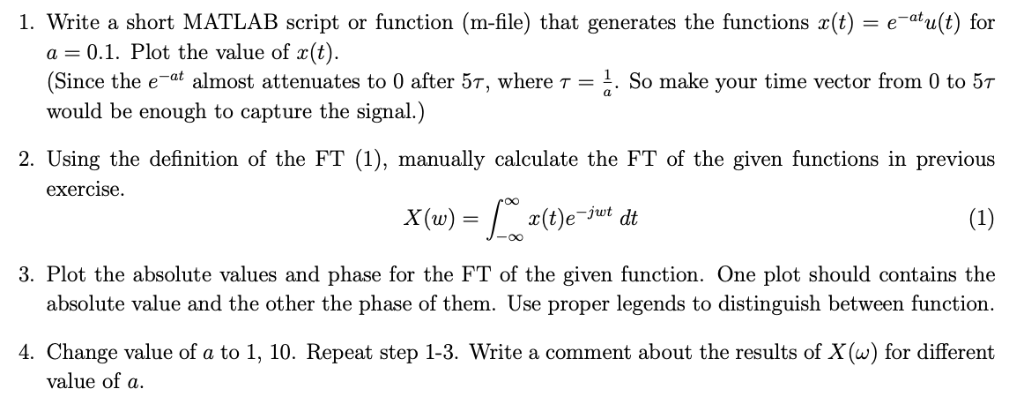Only calculate manually 1. Write a short MATLAB script or function (m-file) that generates the functions (t)eu(t) for a = 0.1. Plot the value of x(t). Since the e-af almost attenuates to 0 after 5T, where T1. So make your time vector from 0 to 5T would be enough to capture the signal.) 2. Using the definition of the FT (1), manually calculate the FT of the given functions in previous exercise 0O x(t)e 3. Plot the absolute values and...

• ### Basic MATLAB : Writing a MATLAB code

Write a MATLAB code to create a graph of two functions on a single plot across an x range of 0 to 2pi :f(x) = sin(2x) - dashed black lineg(x) = 2cos(2x) - green solid lineLabel both the axes ( x and y) and title (use the 'title' command ) the graph " Plot of f(x)=sin(2x) and its derivative. Include a legend that distinguishes the twolines.The number of points that are used to create the lines should be sufficient to...

• ### Write a C code to calculate the following piecewise function using switch. Assume user enters x (...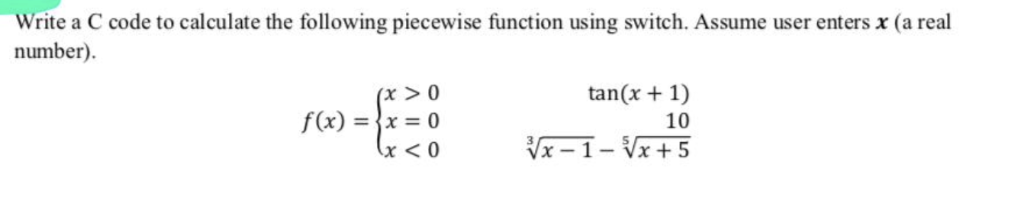Help needed in c program ! Thannx Write a C code to calculate the following piecewise function using switch. Assume user enters x (a real number) tan(x +1) 10 f(x) =,x = 0 x <0 Write a C code to calculate the following piecewise function using switch. Assume user enters x (a real number) tan(x +1) 10 f(x) =,x = 0 x

• ### 3. Use Laplace transforms to solve the following initial value problems. Write the solution (t) f...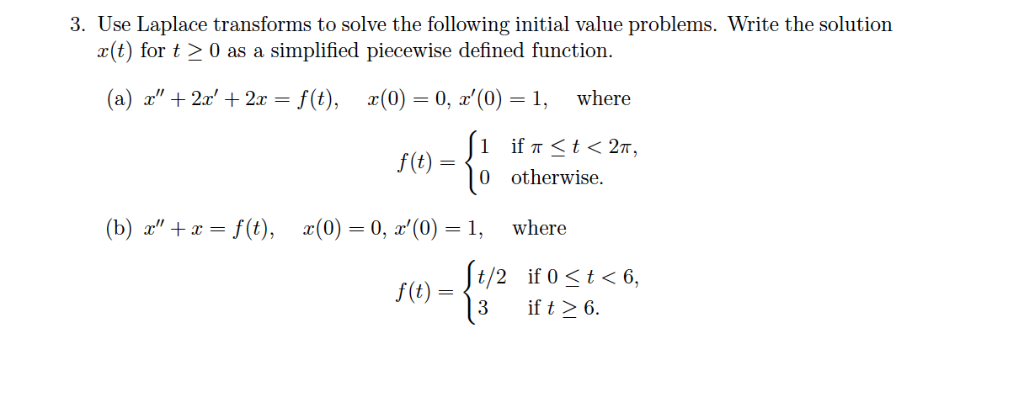3. Use Laplace transforms to solve the following initial value problems. Write the solution (t) for t20 as a simplified piecewise defined function. (a) z', + 2x' + 2x-f(t), x(0-0, z'(0)-i, where f(t)-〈0 otherwise. (b) z', +x-f(t), x(0) 0, z'(0)=1, where t/2 if 0 t< 6, 3 ift26 f(t) 3. Use Laplace transforms to solve the following initial value problems. Write the solution (t) for t20 as a simplified piecewise defined function. (a) z', + 2x' + 2x-f(t), x(0-0, z'(0)-i,...

• ### answer 4, 5, 6, 7 using matlab Homework 10 1. Write the correct first-line syntax for...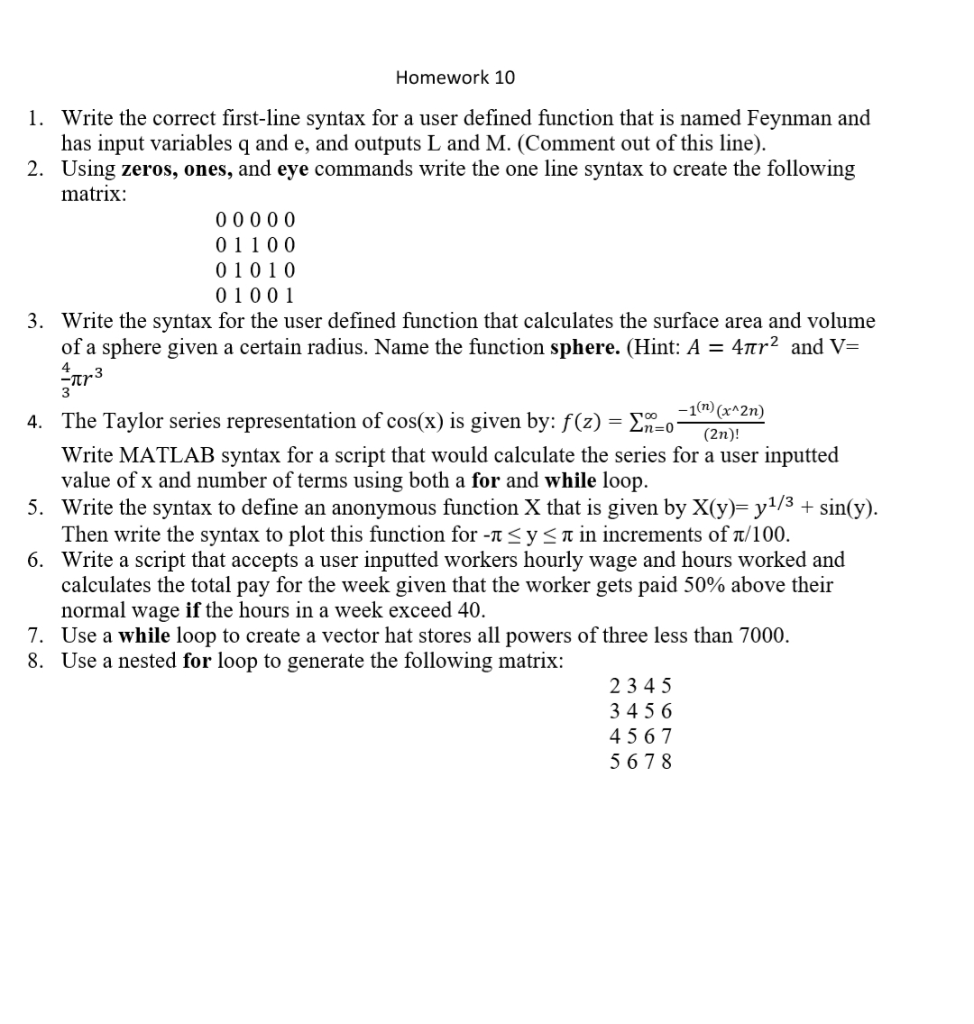answer 4, 5, 6, 7 using matlab Homework 10 1. Write the correct first-line syntax for a user defined function that is named Feynman and has input variables q and e, and outputs L and M. (Comment out of this line). 2. Using zeros, ones, and eye commands write the one line syntax to create the following matrix: 00000 01100 01010 01001 3. Write the syntax for the user defined function that calculates the surface area and volume of a...

• ### Please help me with this MATLAB programming problem! Im coding iin MATLAB2018 if that makes any d...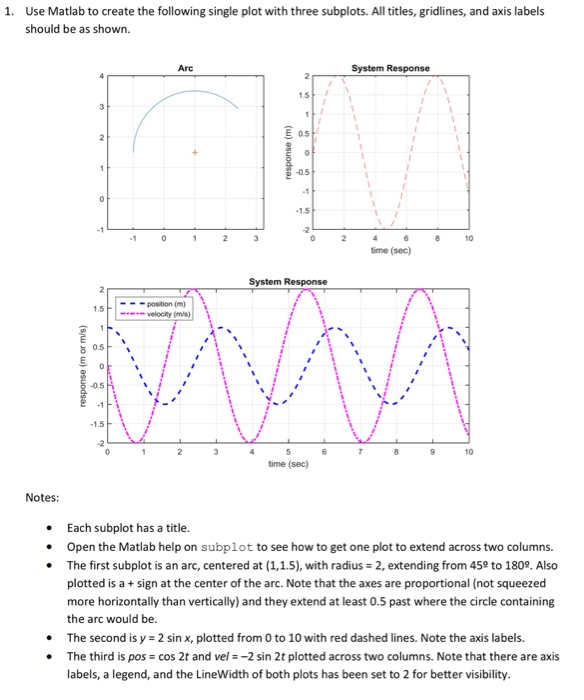Please help me with this MATLAB programming problem! Im coding iin MATLAB2018 if that makes any difference! The first picture is the question i need to answer. The picture below is used as reference to answer the question. The last picture (below) is the into to the problem, and is used as reference. 1. Use Matlab to create the following single plot with three subplots. All titles, gridlines, and axis labels should be as shown. Arc System Response 15 E...

• ### Write a Matlab function for: 1. Root Finding: Calculate the root of the equation f(x)=x^3 −5x^2...

Write a Matlab function for: 1. Root Finding: Calculate the root of the equation f(x)=x^3 −5x^2 +3x−7 Calculate the accuracy of the solution to 1 × 10−10. Find the number of iterations required to achieve this accuracy. Compute the root of the equation with the bisection method. Your program should output the following lines: • Bisection Method: Method converged to root X after Y iterations with a relative error of Z.

• ### Matlab a) Write a MATLAB code that takes the grade letters from the user and provide him/her with the GPA for that seme...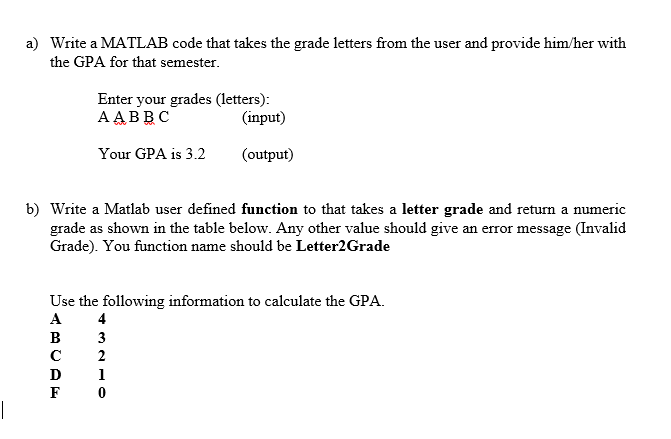Matlab a) Write a MATLAB code that takes the grade letters from the user and provide him/her with the GPA for that semester Enter your grades (letters): AABBC (input) Your GPA is 3.2 output) b) Write a Matlab user defined function to that takes a letter grade and return a numeric grade as shown in the table below. Any other value should give an error message (Invalid Grade). You function name should be Letter2Grade Use the following information to calculate...

• ### i need the required MATLAB script to solve this question please. 1) (20 points) Calculate the following for the function f(x)2x3 a. Calculate the derivative of the function by hand. Write a MATLA...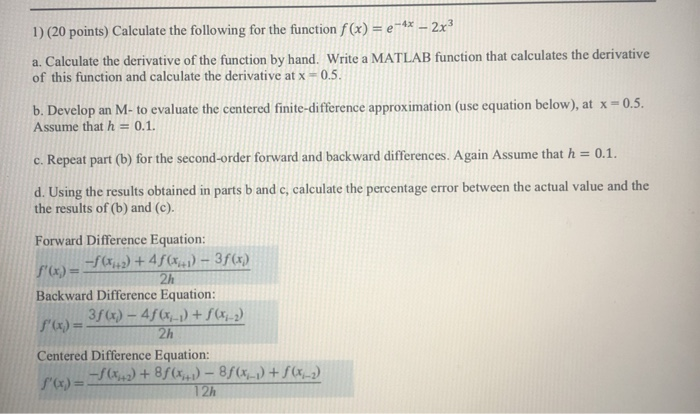i need the required MATLAB script to solve this question please. 1) (20 points) Calculate the following for the function f(x)2x3 a. Calculate the derivative of the function by hand. Write a MATLAB function that calculates the derivative 05. of this function and calculate the derivative at x b. Develop an M- to evaluate the cetered finite-difference approximation (use equation below), at x-o.5. Assume that h 0.1. c Repeat part (b) for the second-order forward and backward differences. Again Assume...

• ### b) (2.5 marks) Write a Matlab user defined function that takes the letter grades (i.e. AABBC) as input and returns...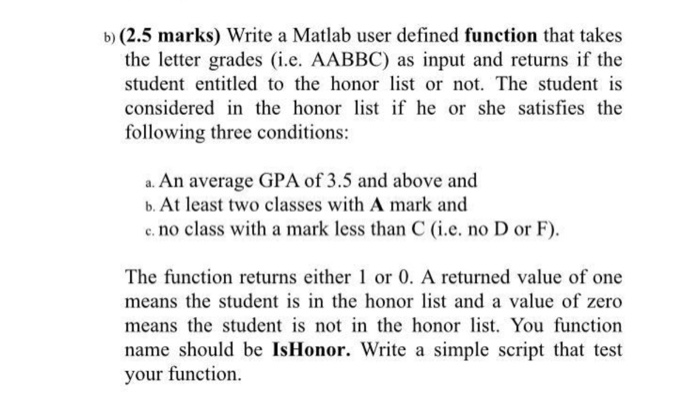b) (2.5 marks) Write a Matlab user defined function that takes the letter grades (i.e. AABBC) as input and returns if the student entitled to the honor list or not. The student is considered in the honor s f he or she satisfies the following three conditions: a. An average GPA of 3.5 and above and b. At least two classes with A mark and e. no class with a mark less than C (i.e. no D or F) The...

Need Online Homework Help?